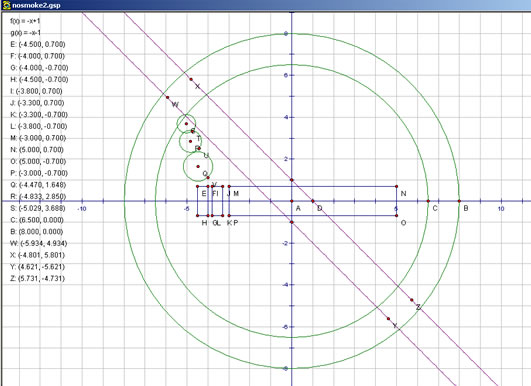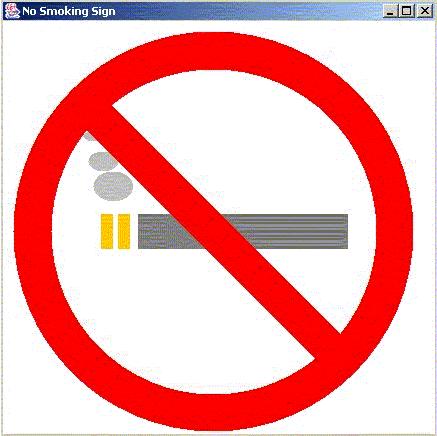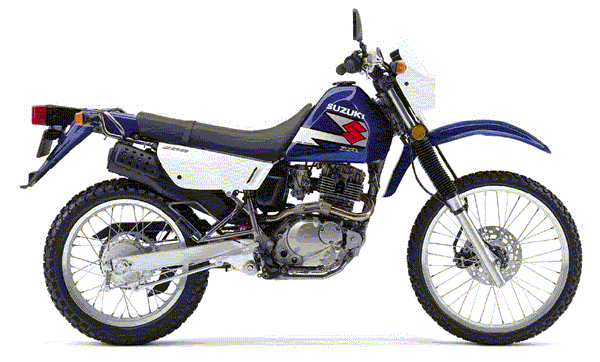Modeling Geometric Objects Using Java

Paul Bouthellier and Saeed Dubas
Department of Mathematics and Computer Science
University of Pittsburgh-Titusville
Titusville, PA 16354
FAX: 814-827-5574
dubas@pitt.edu (Ph: 814-827-5672)
pbouthe@pitt.edu (Ph: 814-827-4432)

Having taught both mathematics and web design, a logical intersection of these two areas is that of computer graphics and mathematical modeling. Constructing mathematical models for 2- and 3-dimensional objects and showing how they are projected onto a computer screen shows computer science students another use of mathematics and gives math students an additional application of their studies.

The study of computer graphics covers a wide range of mathematical topics: Fourier series, Bézier curves, B-splines, graph theory, cross products, quaternions, rotation matrices, and many more. Entire courses are devoted to the mathematical modeling of real-world objects for computer graphics.

In this talk two objects will be constructed using Geometer's Sketchpad and Java. Geometer's Sketchpad is a geometry package which can be used in courses from algebra onwards. Using Java for modeling presents no problems for students as long as enough set-up examples have been given and a basic outline of the Java program is given. What is so nice about using mathematical modeling for computer graphics is that the mathematics used is so diverse that its study serves as an excellent source of practical examples and projects in many undergraduate (and graduate) courses.

Modeling a No-Smoking Sign-Level Algebra

Student's can first model a no-smoking sign by using Geometer's Sketchpad as follows:It is now necessary to translate the points created into: outer and inner circles, rectangles representing a cigarette and its embers, ellipses to represents the puffs of smoke, and a polygon consisting of four points in order to create the strike-through.

The major problem that the students had in this project is in translating the coordinates from sketchpad-which follows the conventions of what is taught in algebra (x increases to the left, y increases in the upwards direction, and the origin (0,0) is the intersection of the x- and y-axes) to the coordinates used in Java (x increases to the left, y increases downwards, and the origin (0,0) is the upper left corner of the application). Translating a [-8,8]x[-8,8] sketchpad coordinate system to a 400x400 (pixel) Java application required the following coordinate transformations:

where (x(sketchpad),y(sketchpad)) and (x(java), y(java)) are the (x,y) coordinates in sketchpad and Java respectively.

After translating the sketchpad points into Java coordinates it became simple to construct the Java application. A portion of the code is shown below:

//outer circle
comp2D.setColor(Color.red);
Ellipse2D.Float outercircle=new Ellipse2D.Float(10F,10F,400F,400F);
comp2D.fill(outercircle);

//inner circle
comp2D.setColor(Color.white);
Ellipse2D.Float innercircle=new Ellipse2D.Float(48F,48F,325F,325F);
comp2D.fill(innercircle);

//Main Cig
comp2D.setColor(Color.gray);
Rectangle2D.Float maincig=new Rectangle2D.Float(135F,193F,210F,35F);
comp2D.fill(maincig);

//Left Ember
comp2D.setColor(Color.orange);
Rectangle2D.Float leftem=new Rectangle2D.Float(98F,193F,12F,35F);
comp2D.fill(leftem);

//Right Ember
comp2D.setColor(Color.orange);
Rectangle2D.Float rightem=new Rectangle2D.Float(115F,193F,12F,35F);
comp2D.fill(rightem);

//Lower Smoke
comp2D.setColor(Color.lightGray);
Ellipse2D.Float lower=new Ellipse2D.Float(90F,150F,40F,30F);
comp2D.fill(lower);

//Middle Smoke
comp2D.setColor(Color.lightGray);
Ellipse2D.Float middle=new Ellipse2D.Float(85F,130F,30F,20F);
comp2D.fill(middle);

//Upper Smoke
comp2D.setColor(Color.lightGray);
Ellipse2D.Float upper=new Ellipse2D.Float(80F,110F,20F,10F);
comp2D.fill(upper);

//Strike Through
comp2D.setColor(Color.red);
GeneralPath strike=new GeneralPath();
strike.moveTo(62F,87F);
strike.lineTo(90F,65F);
strike.lineTo(354F,329F);
strike.lineTo(326F,351F);
strike.closePath();
comp2D.fill(strike);}
}

The resulting Java application is shown below:This project taught students about modeling simple objects with rectangles, circles, ellipses, and polygons. It also required a basic transformation of coordinates. After completing their first projects students are generally interested in going on to more complex projects.

Modeling a Motorcycle-Level Algebra II

Modeling more complex objects require concepts such as curve fitting, rotations, arcs, and angles. I have found that a good mid-level modeling project for students is that of a motorcycle.The hardest part of modeling such a object for students is figuring out where to start. After trying to model the bike as one large polygon, it was discovered that the best approach was to break the problem into pieces. Hence the following pieces were modeled separately: left wheel, right wheel, main column, tire guard, main body, the seat, the engine, the mirror, back wheel connector, and bike logo.

The parts of the motorcycle were modeled as follows:

left wheel, right wheel: circles

main column, main body, engine, seat, wheel connector, and tire guard: polygons and quadratic polynomials

mirror: arc

motorcycle logo: text and a rotation

Once again, as in the nosmoking sign, it was necessary to translate the coordinates from Sketchpad to that of the Java application.

A portion of the resulting code is shown below:

//left wheel

comp2D.setColor(Color.black);
Ellipse2D.Float circlel3=new Ellipse2D.Float(33F,280F,178F,178F);
comp2D.fill(circlel3);

comp2D.setColor(Color.gray);
Ellipse2D.Float circlel2=new Ellipse2D.Float(54F,301F,134F,134F);
comp2D.fill(circlel2);

comp2D.setColor(Color.black);
Ellipse2D.Float circlel1=new Ellipse2D.Float(95F,342F,47F,47F);
comp2D.fill(circlel1);

//logo

comp2D.rotate(-Math.PI/8.0);

Font usethis= new Font("Serif",Font.PLAIN,32);
comp2D.setFont(usethis);
comp2D.setColor(Color.red);
comp2D.drawString("Java 200",155,355);

comp2D.rotate(0.0);

//seat
comp2D.setColor(Color.gray);
GeneralPath seat=new GeneralPath();
seat.moveTo(308F,201F);
seat.lineTo(20F,204F);
seat.lineTo(20F,220F);
seat.lineTo(102F,236F);
seat.lineTo(248F,260F);
seat.closePath();
comp2D.fill(seat);

The resulting Java application is shown below:With minor alterations the code of the application can be turned into an applet so students can put their work on the Internet.

Conclusions

Using mathematical modeling and programs such as Java allow for a wide variety of modeling projects. The applications given here are suitable for precalculus students as a start in modeling. Using art packages for 3-dimensional modeling opens up projects that are suitable for students in many classes at all levels.